Krein condition

A condition in terms of the logarithmic normalized integral(a1)

used to derive non-uniqueness or uniqueness of the moment problem for absolutely continuous probability distributions (cf. also Absolute continuity; Probability distribution). In (a1),is the density function of some distribution functionhaving all moments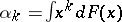,, finite, the integral is taken over the support ofand the argument ofisor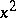, depending on this support.

The general question of interest is: Does the moment sequencedetermineuniquely? If the answer is "yes" , one says that the moment problem has a unique solution, or that the distribution functionis M-determinate. Otherwise, the moment problem has a non-unique solution, or thatis M-indeterminate.

It is essential to note that the quantitydefined in (a1) may "be equal to"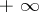.

Hamburger moment problem.

In this problem, the support ofis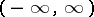, the densityfor alland all moments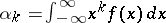,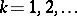, are finite. The values ofbelong to the interval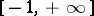.

For this problem the following Krein conditions are used: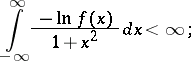(a2)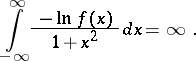(a3)

The following is true:

if (a2) holds, thenis M-indeterminate, i.e. the moment problem has a non-unique solution;

if, in addition to (a3), the Lin condition below is satisfied, thenis M-determinate, i.e. the moment problem has a unique solution.

Here, the following Lin condition is used:is symmetric and differentiable, and for someand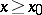,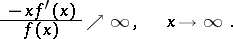(a4)

Stieltjes moment problem.

In this problem, the support ofis the real half-line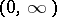, the densityfor all, and all moments,, are finite. In this case the values ofbelong to the interval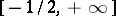.

In this case one uses the following Krein conditions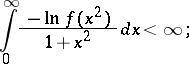(a5)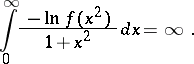(a6)

The following is true:

if (a5) holds, thenis M-indeterminate.

if, in addition to (a6), the Lin condition below is satisfied, thenis M-determinate. Here, the Lin condition is thatbe differentiable and that for some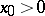and,(a7)

From these four assertions one can derive several interesting results. In particular, one can easily show that the log-normal distribution is M-indeterminate. This fact was discovered by Th.J. Stieltjes in 1894 (in other terms; see [a1], [a3]), and was later given in a probabilistic setting by others, see e.g. [a4].

Examples in probability theory.

Supposeis a random variable with a normal distribution. Then:

the distribution ofis M-indeterminate for all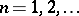;

the distribution of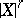is M-determinate for all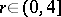;

the distribution ofis M-indeterminate for all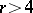. For details (direct constructions and using the Carleman criterion), see [a2]. A proof of this result based on the Krein or Krein–Lin technique is given in [a12].

Letbe a random variable whose distribution is M-determinate. Using the Krein–Lin techniques, one can easily answer questions like: For which values of the real parameterdoes the distribution of the power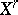and/orbecome M-indeterminate?

Suppose the random variablehas:a normal distribution; an exponential distribution; a gamma-distribution; a logistic distribution; or an inverse Gaussian distribution (cf. also Gauss law). Then in each of these cases the distribution of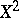is M-determinate, while already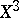has an M-indeterminate distribution, i.e.is the minimal integer power ofdestroying the determinacy of the distribution of. For details see [a12].

A more general problem is to describe classes of functions of random variables (not just powers) preserving or destroying the determinacy of the probability distributions of the given variables.

Generalization.

There is a more general form of the Krein condition, which requires instead of (a2) that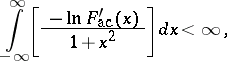whereis the absolutely continuous part of the distribution function, see [a8].

The Krein condition, in conjunction with the Lin condition, is used for absolutely continuous distributions whose densities are positive in both Hamburger and Stieltjes problems. [a7] contains an extension of the Krein condition for indeterminacy as well as a discrete analogue applicable to distributions concentrated on the integers.

The Krein condition can also be used for other purely analytic problems, see [a3] and [a9].

The book [a1] is the basic source describing the progress in the moment problem, providing also an intensive discussion on the Krein condition. For distributions on the real line, this condition was introduced by M.G. Krein in 1944, see [a5]. For recent (1998) developments involving the Krein condition see [a3], [a6], [a7], [a9], [a10]. Several applications of the Krein condition are given in [a11] and [a12].